# Binary Multiplication and Division with Examples

In this tutorial, we will learn about the binary multiplication and division with the help of examples. By Saurabh Gupta Last updated : May 10, 2023

## 1) Binary Multiplication

Binary numbers can be multiplied using two methods,

1. Paper method: Paper method is similar to multiplication of decimal numbers on paper.
2. Computer method: Computer method is used by digital machines to multiply the binary numbers.

However, both these methods follow the same rule of multiplication which is,

```    0 * 0 = 0
0 * 1 = 0
1 * 0 = 0
1 * 1 = 1
```

Note: If two binary numbers are of 'n' bits each in magnitude, then their product can be maximum '2n' bits long in magnitude.

### Long Hand Multiplication/Paper Method

The long Hand Multiplication technique is similar to decimal multiplication that we do on paper.

In this technique, we multiply the multiplicand with each bit of multiplier and add the partial products together to obtain the result.

We scan the multiplier from the RHS, if the multiplier bit is 1 then we copy the whole multiplicand in the partial product and if the multiplier bit is 0, we replace all the bits of the multiplicand with 0 in the partial product.

#### Solution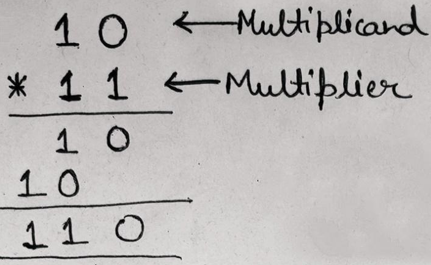Therefore, the result is (10)2 * (11)2 = (110)2

Verification:

We can verify our result by converting the binary numbers to respective decimal numbers and multiplying them to get the result.

Here, (10)2 = (2)10, (11)2 = (3)10 and (110)2 = (6)10. When we will multiply 2 and 3, we will get the product as 6, which we are getting by multiplication of binary numbers. Hence our solution is correct.

#### Solution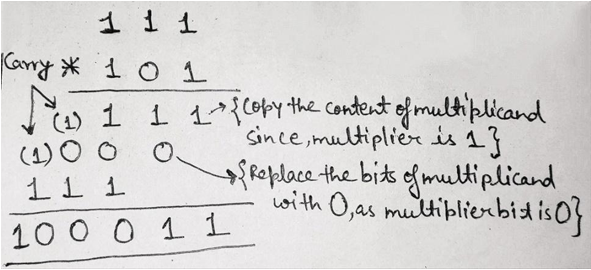Therefore, the result is (111)2 * (101)2 = (100011)2

#### Solution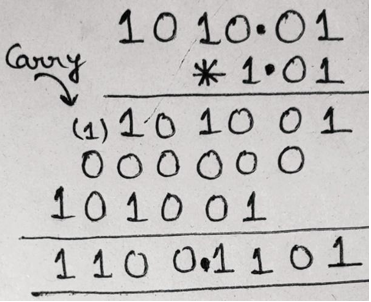Therefore, the result is (1010.01)2 * (1.01)2 = (1100.1101)2

## 2) Binary Division

Like binary multiplication, division of binary numbers can also be done in two ways which are:

1. Paper Method: Paper Method division of binary numbers is similar to decimal division.
2. Computer Method: Computer Method is used by the digital devices where they make use of 2's complement of a number to subtract the numbers during division.

### Long Hand Division Method/Paper Method

In binary division, there are only two possibilities either 0 or 1. If the divisor goes into the dividend then quotient will be 1 and if the divisor doesn’t then the quotient will be 0. Similar, to decimal division, we will subtract divisor from the dividend and the result will be appended by the next bit in the dividend. This process repeats until all the bits of the dividend are considered.

#### Solution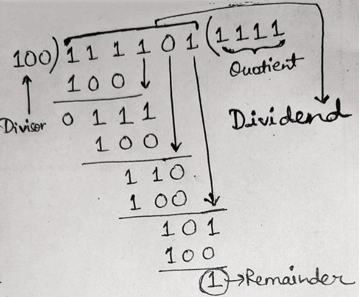Therefore, the result is (111101)2 / (100)2 gives (1111)2 (Quotient) and 1 (Remainder)

#### Solution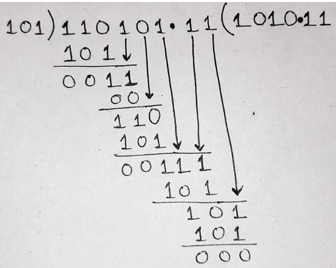Therefore, the result is (110101.11)2 / (101)2 gives (1010.11)2.

Verification:

We can verify our result by converting the binary numbers to respective decimal numbers and then dividing the dividend by the divisor to get the result.

Here, (110101.11)2 = (53.75)10, (101)2 = (5)10 and (1010.11)2 = (10.75)10. When we will divide 53.75 by 5, we will get the result as 10.75, which we are getting by doing division of binary numbers. Hence our solution is correct.

#### Solution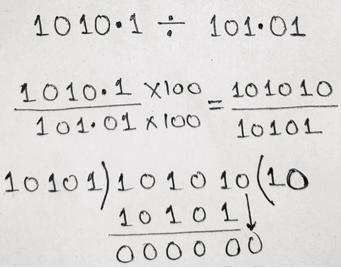Therefore, the result is (1010.1)2 / (101.01)2 gives (10)2.